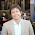## Sunday, February 16, 2014

### Frenkel on Platonism, Tegmark, and simulation

Math professor Edward Frenkel writes in the NY Times:
Indeed, there may be. In a recent paper, “Constraints on the Universe as a Numerical Simulation,” the physicists Silas R. Beane, Zohreh Davoudi and Martin J. Savage outline a possible method for detecting that our world is actually a computer simulation. Physicists have been creating their own computer simulations of the forces of nature for years — on a tiny scale, the size of an atomic nucleus. They use a three-dimensional grid to model a little chunk of the universe; then they run the program to see what happens. This way, they have been able to simulate the motion and collisions of elementary particles.

But these computer simulations, Professor Beane and his colleagues observe, generate slight but distinctive anomalies — certain kinds of asymmetries. Might we be able to detect these same distinctive anomalies in the actual universe, they wondered? In their paper, they suggest that a closer look at cosmic rays, those high-energy particles coming to Earth’s atmosphere from outside the solar system, may reveal similar asymmetries. If so, this would indicate that we might — just might — ourselves be in someone else’s computer simulation.
This is science fiction. The fact is that we do not have the know-how to mathematically simulate the universe. We can simulate particular experiments, but any simulation we would produce would have some differences with reality. If we lived in a simulation with the same shortcomings, then they would be detectable.

Part of the belief in quantum computing is based on the fact that we cannot simulate the quantum world. Feynman once gave a lecture where he speculated that we might need a quantum computer for an effective simulation.
An argument can also be made that mathematical ideas are objective and exist independently from the human mind — many mathematicians adhere to this view, called mathematical Platonism.

Yet Tegmark’s take is quite different from — and in some ways inconsistent with — Platonism. Math is so effective in describing the world, he says, because physical reality is a mathematical structure. He calls it the Mathematical Universe Hypothesis (M.U.H.). What exactly this means is a big question, which is never fully answered. Mr. Tegmark’s argument is that all physical properties of an electron, say, can be described mathematically; therefore, to him, an electron is itself a mathematical structure — as is everything else, including us.
Mathematical Platonism is reasonable, in my opinion, but Tegmark is saying something different.

Yes, our theories are mathematical, and they predict experiments well, but Tegmark wants to make an ontological argument that the physics is the math. If two distant electrons are entangled, then it is impossible to give a mathematical description of one electron independent of the other one. The usual way out of this paradox is to day that there is a mathematical action-at-a-distance, but not a physical one. To me, that says that the physics is not the math.

There are alternative explanations, such as saying that there is a physical nonlocality that is not directly observable. To me, this is unsatisfactory as it is like believing in psychic powers that can never be demonstrated in a controlled way, or believing in one of the unobservable multiverses.

Thus I disagree with Tegmark than an individual electron can be described as a mathematical structure. It cannot. We have a good mathematical description of the measurement possibilities of two entangled electrons jointly, but we cannot identify one of them individually with a mathematical structure. Attempts to do so have led to hidden variable theories, and they have all failed.

Update: Mad Max comments below that Frenkel has misrepresented his views, and concedes the point that entanglement issues prevent an individual electron from being equated with a mathematical structure.

I think that this is an important point. Some of Tegmark's critics have questioned whether there is any content to the claim that the universe is mathematical, because no one knows what a non-mathematical universe would look like. If he is now conceding that an isolated but entangled electron is non-mathematical, then that is an example of a physical object that is not a mathematical structure. It seems to me that if an electron can be a non-mathematical object, then so can the universe. Tegmark would say that the electrons and other objects fit together to make a giant mathematical structure, even if some of the physical components are not.

#### 1 comment:

1.Thanks Roger for bringing up one of my favorite topics: entanglement!
In my book (http://mathematicaluniverse.org), I never say what you attribute to me above (that an individual electron can be described as a mathematical structure). Your point about entanglement is correct: the quantum wavefunction describes the joint state of *all* particles (and everything else). Erwin Schrödinger pointed this out in the the 1920s. The mathematical universe hypothesis I explore in the book is that it's our entire physical reality (not a particle individually) that's a mathematical structure.